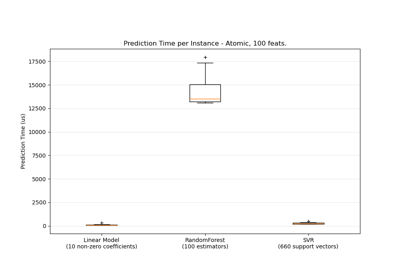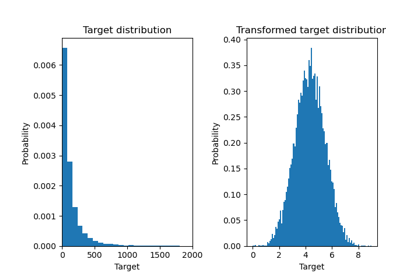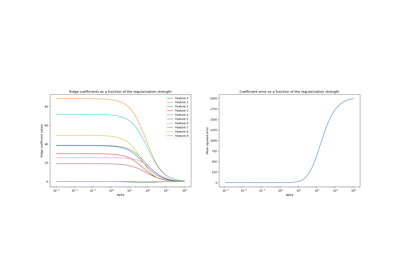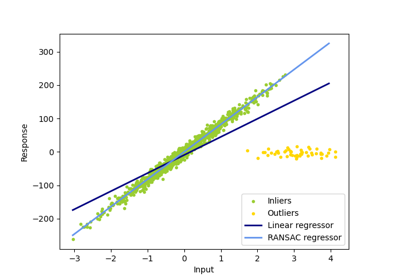# sklearn.datasets.make_regression¶

sklearn.datasets.make_regression(n_samples=100, n_features=100, n_informative=10, n_targets=1, bias=0.0, effective_rank=None, tail_strength=0.5, noise=0.0, shuffle=True, coef=False, random_state=None)[source]

Generate a random regression problem.

The input set can either be well conditioned (by default) or have a low rank-fat tail singular profile. See make_low_rank_matrix for more details.

The output is generated by applying a (potentially biased) random linear regression model with n_informative nonzero regressors to the previously generated input and some gaussian centered noise with some adjustable scale.

Read more in the User Guide.

Parameters: n_samples : int, optional (default=100) The number of samples. n_features : int, optional (default=100) The number of features. n_informative : int, optional (default=10) The number of informative features, i.e., the number of features used to build the linear model used to generate the output. n_targets : int, optional (default=1) The number of regression targets, i.e., the dimension of the y output vector associated with a sample. By default, the output is a scalar. bias : float, optional (default=0.0) The bias term in the underlying linear model. effective_rank : int or None, optional (default=None) if not None: The approximate number of singular vectors required to explain most of the input data by linear combinations. Using this kind of singular spectrum in the input allows the generator to reproduce the correlations often observed in practice. if None: The input set is well conditioned, centered and gaussian with unit variance. tail_strength : float between 0.0 and 1.0, optional (default=0.5) The relative importance of the fat noisy tail of the singular values profile if effective_rank is not None. noise : float, optional (default=0.0) The standard deviation of the gaussian noise applied to the output. shuffle : boolean, optional (default=True) Shuffle the samples and the features. coef : boolean, optional (default=False) If True, the coefficients of the underlying linear model are returned. random_state : int, RandomState instance or None (default) Determines random number generation for dataset creation. Pass an int for reproducible output across multiple function calls. See Glossary. X : array of shape [n_samples, n_features] The input samples. y : array of shape [n_samples] or [n_samples, n_targets] The output values. coef : array of shape [n_features] or [n_features, n_targets], optional The coefficient of the underlying linear model. It is returned only if coef is True.

## Examples using sklearn.datasets.make_regression¶Prediction LatencyEffect of transforming the targets in regression modelPlot Ridge coefficients as a function of the L2 regularizationRobust linear model estimation using RANSACLasso on dense and sparse dataHuberRegressor vs Ridge on dataset with strong outliers